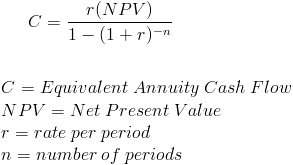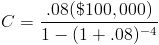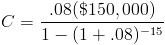# Equivalent Annual AnnuityThe equivalent annual annuity formula is used in capital budgeting to show the net present value of an investment as a series of equal cash flows for the length of the investment. The net present value(NPV) formula shows the present value of an investment that has uneven cash flows. When comparing two different investments using the net present value method, the length of the investment (n) is not taken into consideration. An investment with a 15 year term may show a higher NPV than an investment with a 4 year term. By showing the NPV as a series of cash flows, the equivalent annual annuity formula provides a way to factor in the length of an investment.

## How is the Equivalent Annual Annuity Formula Useful?

An example of how the equivalent annual annuity formula may be useful is comparing two new projects where one project has a 15 year term and the other has a 4 year term. Assume that both projects have the same NPV. The 4 year project will receive the return sooner so it will show a higher cash flow when using the equivalent annual annuity formula. In real life, comparing two investments will not always be so obvious and the formula should be applied.

Another way of explaining the usefulness of the equivalent annual annuity formula is that an investment with a shorter life span can be reinvested and the earnings on the reinvestment is not taken into consideration when using the NPV formula. The equivalent annual annuity formula provides a comparison relative to time which eliminates the need for considering reinvestment with the same earnings as the current investment.

## How is the Equivalent Annual Annuity Formula Derived?

The equivalent annual annuity formula uses the annuity payment formula for when present value is given. Net present value replaces present value to give relevance to the use of the equivalent annual annuity formula.

## Example of the Equivalent Annual Annuity Formula

Using the prior example of comparing one project with a 4 year term and another project with a 15 year term, the NPV of the 4 year project is \$100,000 and the NPV of the 15 year project is \$150,000. The rate used for both is 8%. Putting the variables of the 4 year project in the equivalent annual annuity formula showswhich returns an equivalent annual annuity of \$30,192.08.

Putting the variables of the 15 year project into the equivalent annual annuity formula showswhich returns an equivalent annual annuity of \$17,524.43.

Comparing these two projects, the 4 year project will return a higher amount relative to the time of the investment. Although the 15 year project has a higher NPV, the 4 year project can be reinvested and have additional earnings for the 11 years that remain on the 15 year project.

New to Finance?